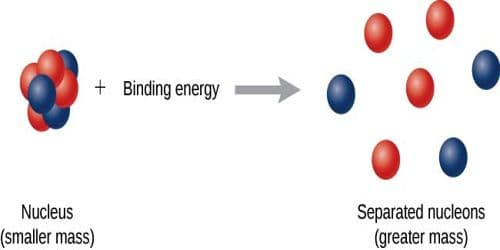# Binding Energy per Nucleon

Binding Energy per Nucleon

We know, each atom of all the elements, except hydrogen, is composed of protons and neutrons. These are called nucleons. The total number of nucleons is called the mass number. It is represented by μ. Now we will discuss what is binding energy per nucleon.

The ratio of the binding energy of the nucleus and the mass number is called the binding energy of each nucleon. Binding energy per nucleon is determined by dividing the total binding energy by mass number. It is also called the avenge binding energy.

Binding energy per nucleon = [total binding energy / total number of nucleons].

(B.E.)/A = (Δmc2/A) MeV/nucleon.

Average binding energy or binding energy per nucleon is a significant quantity. Binding energy per nucleon of a nucleus depends on the mass number. As the mass number changes average binding energy also changes-

Mathematical Example

Find the (i) mass defect, (ii) binding energy and (iii) binding energy per neutron for a helium (2He4) nucleus. [Mass of a proton = 100728 a.m.u., mass of a neutron = 1.00876 a.m.u. and 1 a.m.u. = 931 MeV].

We know, there are two protons and two neutrons in a helium nucleus. Now, mass of two protons = 2 x 1.00728 = 2.014856 a.m.u.

Mass of two neutrons = 2 x 1.00867 = 2.01734 a.m.u.

Their total mass = 2.014556 + 2.01734 = 4.03190 a.m.u.

But actual mass of a helium nucleus = 4.00276 a.m.u.

(i) Mass defect, Δm = 4.03190 – 4.00276 = 0.02914 a.m.u.

(ii) Binding energy = Δm x 931 = 0.02914 x 931 = 27.129 MeV

(iii) Number of nucleon in helium nucleus = 4

Binding energy per nucleon = B.E./4 = 27.127/4 = 6.782 MeV.